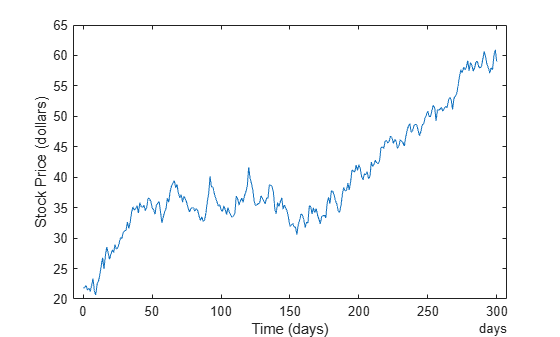## Detrending Data

### Introduction

The function `detrend` subtracts the mean or a best-fit line (in the least-squares sense) from your data. If your data contains several data columns, `detrend` treats each data column separately.

Removing a trend from the data enables you to focus your analysis on the fluctuations in the data about the trend. A linear trend typically indicates a systematic increase or decrease in the data. A systematic shift can result from sensor drift, for example. While trends can be meaningful, some types of analyses yield better insight once you remove trends.

Whether it makes sense to remove trend effects in the data often depends on the objectives of your analysis.

### Remove Linear Trends from Data

This example shows how to remove a linear trend from daily closing stock prices to emphasize the price fluctuations about the overall increase. If the data does have a trend, detrending it forces its mean to zero and reduces overall variation. The example simulates stock price fluctuations using a distribution taken from the `gallery` function.

Create a simulated data set and compute its mean. `sdata` represents the daily price changes of a stock.

```rng(20) t = 0:300; dailyFluct = randn(size(t)); sdata = cumsum(dailyFluct) + 20 + t/100;```

Find the average of the data.

`mean(sdata)`
```ans = 41.5155 ```

Plot and label the data. Notice the systematic increase in the stock prices that the data displays.

```figure plot(t,sdata); legend('Original Data','Location','northwest'); xlabel('Time (days)'); ylabel('Stock Price (dollars)');```Apply `detrend`, which performs a linear fit to `sdata` and then removes the trend from it. Subtracting the output from the input yields the computed trend line.

```detrend_sdata = detrend(sdata); trend = sdata - detrend_sdata;```

Find the average of the detrended data.

`mean(detrend_sdata)`
```ans = -8.0025e-15 ```

As expected, the detrended data has a mean very close to 0.

Display the results by adding the trend line, the detrended data, and its mean to the graph.

```hold on plot(t,trend,':r') plot(t,detrend_sdata,'m') plot(t,zeros(size(t)),':k') legend('Original Data','Trend','Detrended Data',... 'Mean of Detrended Data','Location','northwest') xlabel('Time (days)'); ylabel('Stock Price (dollars)');```